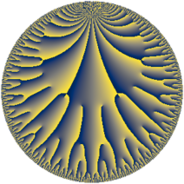# Properties

 Label 75.22.aLevel $75$ Weight $22$ Character orbit 75.a Rep. character $\chi_{75}(1,\cdot)$ Character field $\Q$ Dimension $66$ Newform subspaces $14$ Sturm bound $220$ Trace bound $2$

# Related objects

## Defining parameters

 Level: $$N$$ $$=$$ $$75 = 3 \cdot 5^{2}$$ Weight: $$k$$ $$=$$ $$22$$ Character orbit: $$[\chi]$$ $$=$$ 75.a (trivial) Character field: $$\Q$$ Newform subspaces: $$14$$ Sturm bound: $$220$$ Trace bound: $$2$$ Distinguishing $$T_p$$: $$2$$

## Dimensions

The following table gives the dimensions of various subspaces of $$M_{22}(\Gamma_0(75))$$.

Total New Old
Modular forms 216 66 150
Cusp forms 204 66 138
Eisenstein series 12 0 12

The following table gives the dimensions of the cuspidal new subspaces with specified eigenvalues for the Atkin-Lehner operators and the Fricke involution.

$$3$$$$5$$FrickeDim.
$$+$$$$+$$$$+$$$$15$$
$$+$$$$-$$$$-$$$$18$$
$$-$$$$+$$$$-$$$$17$$
$$-$$$$-$$$$+$$$$16$$
Plus space$$+$$$$31$$
Minus space$$-$$$$35$$

## Trace form

 $$66 q + 1598 q^{2} + 68549728 q^{4} - 105225318 q^{6} + 559598932 q^{7} + 6582404412 q^{8} + 230127770466 q^{9} + O(q^{10})$$ $$66 q + 1598 q^{2} + 68549728 q^{4} - 105225318 q^{6} + 559598932 q^{7} + 6582404412 q^{8} + 230127770466 q^{9} - 140550939936 q^{11} + 212790629772 q^{12} + 476755719848 q^{13} + 5951926495932 q^{14} + 74654476857140 q^{16} + 2895711111680 q^{17} + 5571881472798 q^{18} + 20854110608092 q^{19} - 53270783960796 q^{21} - 305003876572940 q^{22} + 332917865015616 q^{23} - 146507777465724 q^{24} - 576566152075128 q^{26} + 5134394163382324 q^{28} - 4640335547701836 q^{29} + 1051949236026996 q^{31} - 11777670119768948 q^{32} - 5075328150422316 q^{33} + 5320941144924676 q^{34} + 239018122283192928 q^{36} + 43291387140114432 q^{37} + 1762108332088064 q^{38} - 41884285798323612 q^{39} - 8589059150896524 q^{41} - 276597377309387484 q^{42} + 337242287299800512 q^{43} - 518361176774084976 q^{44} - 520177620234876064 q^{46} + 41641218071661944 q^{47} + 757307119626960768 q^{48} + 6307843855416529270 q^{49} - 456369712891637472 q^{51} + 3678055801729764120 q^{52} - 4772255569432856824 q^{53} - 366897997392664518 q^{54} + 8305011149862147480 q^{56} - 1235155990591987416 q^{57} - 13003977897019306552 q^{58} - 9360986021400428352 q^{59} + 16978528007485279200 q^{61} + 23551546864273794264 q^{62} + 1951200826913859732 q^{63} + 79397858551386240332 q^{64} + 5266431451152350856 q^{66} + 48974675829821969080 q^{67} + 70650292973404157056 q^{68} - 31248085931695120968 q^{69} + 19836002127692491872 q^{71} + 22951425024835177212 q^{72} - 62504040788772941884 q^{73} - 320787328791694921068 q^{74} + 356693975412702702128 q^{76} + 562671241634060126784 q^{77} - 243727291343685212820 q^{78} - 454274786635617948080 q^{79} + 802405920297757300866 q^{81} + 861244093915636154684 q^{82} - 71078281851982135896 q^{83} - 304707361620478730472 q^{84} - 901966438206279205596 q^{86} + 674271097049404924308 q^{87} - 678426143664569738508 q^{88} - 2210353435062926891148 q^{89} - 1359502168448881585004 q^{91} + 4401063710813395972032 q^{92} + 103186520604806078808 q^{93} - 2381241412886494240592 q^{94} - 3754707195708424128996 q^{96} + 1109641272603148372380 q^{97} - 4335613966611832465538 q^{98} - 490070824914732738336 q^{99} + O(q^{100})$$

## Decomposition of $$S_{22}^{\mathrm{new}}(\Gamma_0(75))$$ into newform subspaces

Label Dim $A$ Field CM Traces A-L signs $q$-expansion
$a_{2}$ $a_{3}$ $a_{5}$ $a_{7}$ 3 5
75.22.a.a $1$ $209.608$ $$\Q$$ None $$-1728$$ $$59049$$ $$0$$ $$-538429808$$ $-$ $+$ $$q-12^{3}q^{2}+3^{10}q^{3}+888832q^{4}+\cdots$$
75.22.a.b $1$ $209.608$ $$\Q$$ None $$-544$$ $$-59049$$ $$0$$ $$-1277698380$$ $+$ $+$ $$q-544q^{2}-3^{10}q^{3}-1801216q^{4}+\cdots$$
75.22.a.c $1$ $209.608$ $$\Q$$ None $$2844$$ $$59049$$ $$0$$ $$-363303920$$ $-$ $+$ $$q+2844q^{2}+3^{10}q^{3}+5991184q^{4}+\cdots$$
75.22.a.d $2$ $209.608$ $$\Q(\sqrt{649})$$ None $$-666$$ $$-118098$$ $$0$$ $$-679896112$$ $+$ $+$ $$q+(-333-\beta )q^{2}-3^{10}q^{3}+(589618+\cdots)q^{4}+\cdots$$
75.22.a.e $3$ $209.608$ $$\mathbb{Q}[x]/(x^{3} - \cdots)$$ None $$-803$$ $$-177147$$ $$0$$ $$1577598316$$ $+$ $+$ $$q+(-268+\beta _{1})q^{2}-3^{10}q^{3}+(2238318+\cdots)q^{4}+\cdots$$
75.22.a.f $3$ $209.608$ $$\mathbb{Q}[x]/(x^{3} - \cdots)$$ None $$-702$$ $$177147$$ $$0$$ $$2072418204$$ $-$ $+$ $$q+(-234-\beta _{1})q^{2}+3^{10}q^{3}+(1748076+\cdots)q^{4}+\cdots$$
75.22.a.g $3$ $209.608$ $$\mathbb{Q}[x]/(x^{3} - \cdots)$$ None $$2300$$ $$-177147$$ $$0$$ $$-465666872$$ $+$ $+$ $$q+(767+\beta _{1})q^{2}-3^{10}q^{3}+(421908+\cdots)q^{4}+\cdots$$
75.22.a.h $4$ $209.608$ $$\mathbb{Q}[x]/(x^{4} - \cdots)$$ None $$897$$ $$236196$$ $$0$$ $$234577504$$ $-$ $+$ $$q+(224+\beta _{1})q^{2}+3^{10}q^{3}+(-290854+\cdots)q^{4}+\cdots$$
75.22.a.i $6$ $209.608$ $$\mathbb{Q}[x]/(x^{6} - \cdots)$$ None $$-92$$ $$354294$$ $$0$$ $$-1327143454$$ $-$ $-$ $$q+(-15+\beta _{1})q^{2}+3^{10}q^{3}+(731822+\cdots)q^{4}+\cdots$$
75.22.a.j $6$ $209.608$ $$\mathbb{Q}[x]/(x^{6} - \cdots)$$ None $$92$$ $$-354294$$ $$0$$ $$1327143454$$ $+$ $+$ $$q+(15-\beta _{1})q^{2}-3^{10}q^{3}+(731822+\cdots)q^{4}+\cdots$$
75.22.a.k $8$ $209.608$ $$\mathbb{Q}[x]/(x^{8} - \cdots)$$ None $$-666$$ $$472392$$ $$0$$ $$-134034472$$ $-$ $+$ $$q+(-83-\beta _{1})q^{2}+3^{10}q^{3}+(1335201+\cdots)q^{4}+\cdots$$
75.22.a.l $8$ $209.608$ $$\mathbb{Q}[x]/(x^{8} - \cdots)$$ None $$666$$ $$-472392$$ $$0$$ $$134034472$$ $+$ $-$ $$q+(83+\beta _{1})q^{2}-3^{10}q^{3}+(1335201+\cdots)q^{4}+\cdots$$
75.22.a.m $10$ $209.608$ $$\mathbb{Q}[x]/(x^{10} - \cdots)$$ None $$-645$$ $$590490$$ $$0$$ $$-115357290$$ $-$ $-$ $$q+(-65+\beta _{1})q^{2}+3^{10}q^{3}+(1004337+\cdots)q^{4}+\cdots$$
75.22.a.n $10$ $209.608$ $$\mathbb{Q}[x]/(x^{10} - \cdots)$$ None $$645$$ $$-590490$$ $$0$$ $$115357290$$ $+$ $-$ $$q+(65-\beta _{1})q^{2}-3^{10}q^{3}+(1004337+\cdots)q^{4}+\cdots$$

## Decomposition of $$S_{22}^{\mathrm{old}}(\Gamma_0(75))$$ into lower level spaces

$$S_{22}^{\mathrm{old}}(\Gamma_0(75)) \cong$$ $$S_{22}^{\mathrm{new}}(\Gamma_0(1))$$$$^{\oplus 6}$$$$\oplus$$$$S_{22}^{\mathrm{new}}(\Gamma_0(3))$$$$^{\oplus 3}$$$$\oplus$$$$S_{22}^{\mathrm{new}}(\Gamma_0(5))$$$$^{\oplus 4}$$$$\oplus$$$$S_{22}^{\mathrm{new}}(\Gamma_0(15))$$$$^{\oplus 2}$$$$\oplus$$$$S_{22}^{\mathrm{new}}(\Gamma_0(25))$$$$^{\oplus 2}$$